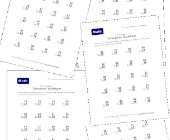Help kids learn math with our collection of subtraction worksheets for kids that are perfect for any math lesson plans. This section of math worksheets is geared towards helping children learn subtraction. Our subtraction worksheets include printable pages of problems with borrowing, without borrowing, borrowing across zeros, 2 digits, 3 digits, 4 digits and more. Check out each group of subtraction worksheets below and print out your copies to help your kids learn math.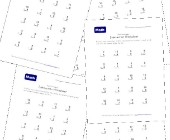Easy Subtraction Worksheets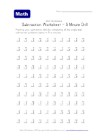Five Minute Subtraction Drill Worksheet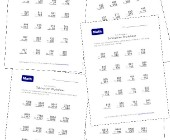Math Help Worksheets - Practice Subtraction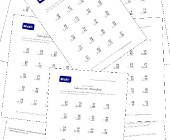Math Worksheets - Two Digit Subtraction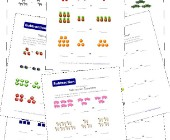Picture Subtraction Worksheets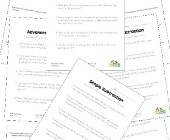Subtraction Word Problems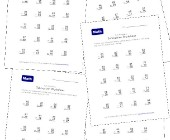Subtraction Worksheets - With Borrowing